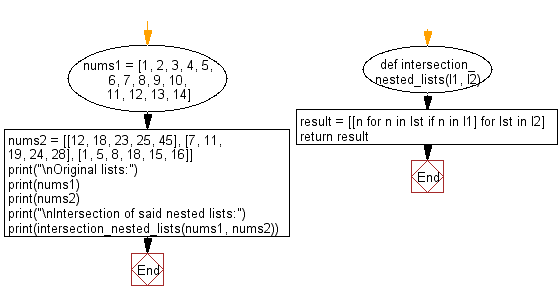﻿ Python: Find the nested lists elements which are present in another list - w3resource# Python: Find the nested lists elements which are present in another list

## Python List: Exercise - 121 with Solution

Write a Python program to find the nested lists elements which are present in another list.

Sample Solution:

Python Code:

``````def intersection_nested_lists(l1, l2):
result = [[n for n in lst if n in l1] for lst in l2]
return result
nums1 = [1, 2, 3, 4, 5, 6, 7, 8, 9, 10, 11, 12, 13, 14]
nums2 = [[12, 18, 23, 25, 45], [7, 11, 19, 24, 28], [1, 5, 8, 18, 15, 16]]
print("\nOriginal lists:")
print(nums1)
print(nums2)
print("\nIntersection of said nested lists:")
print(intersection_nested_lists(nums1, nums2))
```
```

Sample Output:

```Original lists:
[1, 2, 3, 4, 5, 6, 7, 8, 9, 10, 11, 12, 13, 14]
[[12, 18, 23, 25, 45], [7, 11, 19, 24, 28], [1, 5, 8, 18, 15, 16]]

Intersection of said nested lists:
[, [7, 11], [1, 5, 8]]
```

Flowchart:## Visualize Python code execution:

The following tool visualize what the computer is doing step-by-step as it executes the said program:

Python Code Editor:

Have another way to solve this solution? Contribute your code (and comments) through Disqus.

What is the difficulty level of this exercise?

Test your Python skills with w3resource's quiz

﻿

## Python: Tips of the Day

Floor Division:

When we speak of division we normally mean (/) float division operator, this will give a precise result in float format with decimals.

For a rounded integer result there is (//) floor division operator in Python. Floor division will only give integer results that are round numbers.

```print(1000 // 300)
print(1000 / 300)```

Output:

```3
3.3333333333333335```